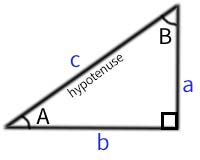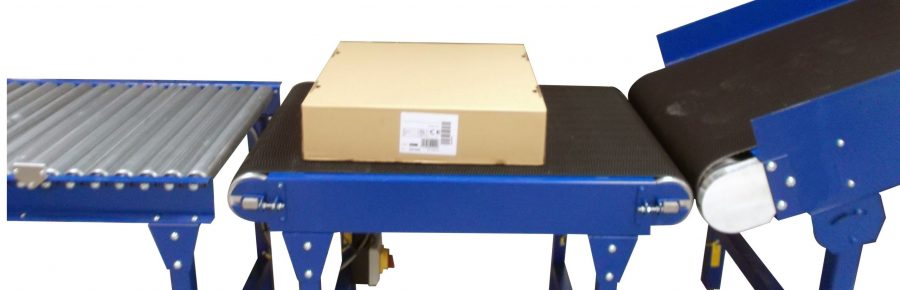Home » Articles » conveyors » Incline Conveyor Calculators

# Incline Conveyor Calculators

posted in: conveyors

## Incline Conveyor Calculators

When planning for a conveyor system design, there are numerous factors that need to be taken into account. These calculators are designed to assist in working out safe incline angles, conveyor heights, and other useful measurements. The box tumbling calculator gives the maximum angle at which products can safely be transported without tumbling.

### Incline Conveyor Length Calculator

When using a grip faced belt the maximum incline angle is 23°

Show values to . . . significant figures.

 edge a = units edge b = units edge c = units angle A = degrees angle B = degrees Total area = square units## Box Tumbling## Motor Power Calculation

Belt Pull Result:

Motor Power Result:

## Calculate Amps